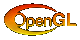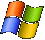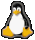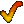"There's a saying that states: 'The road to hell is paved with good intentions'. There's hardly anything that better describes communism than that. All good hopes and dreams of justice and equality have ended in oppression and dictatorship."
- Peter Eriksson, Green Party of Sweden

Framework 3 (Last updated: February 6, 2017)
Framework 2 (Last updated: October 8, 2006)
Framework (Last updated: October 8, 2006)
Libraries (Last updated: September 16, 2004)
Really old framework (Last updated: September 16, 2004)
Metaballs
Sunday, January 2, 2005 | PermalinkExecutableSource code
MetaBalls.zip (808 KB)

Required:
GL_ARB_shader_objects
GL_ARB_vertex_shader
GL_ARB_fragment_shader
GL_ARB_shading_language_100
This demo implements the marching cubes algorithm for constructing a polygonal model from an isosurface. The isosurface chosen here is a classical metaballs setup. Metaballs are defined by field function that's the sum of R2 / ((ball.x - x)2 + (ball.y - y)2 + (ball.z - z)2) for all balls. The surface is defined to be where the sum is one.

The marching cubes algorithm constructs the isosurface by intersecting the space with cubes (also referred to as cells). At each vertex point the field function is evaluated. An edge on a cube is known to intersect the surface if one has a value above one, or in my implementation, is positive (I subtract one so I can just use the sign bits, which speeds things up). At each edge that intersects the surface an approximate intersection point is computed by interpolating between the edge points. As there are 8 corners in a cube that are either above or below the surface there are 28 = 256 possible ways for the surface to intersect the cube. These are stored a lookup table for quick assembly. Many implementations on the web evaluate the vertices directly for each cube and doesn't evaluate the surface topology. This is simpler, but means a lot of computations are repeated. My implementation however evaluates the full topology with both an index array and vertex array, which is faster and requires less data to send to the graphic card, but it uses a bit more memory and requires a bit more advanced data structure. The topology may also be useful for other tasks such as computing smooth surface normals. I'm evaluating the normals directly from the field function however as this was both faster and gave higher quality normals.

The main bottleneck in this process is evaluating the field function. To speed things up a bit I implemented 3DNow and SSE versions of it as well. It should choose the most appropriate for your system as default. You can change what field function to use on the F1 menu.

As a final output of all this I apply a water-esque shader on the surface.

This demo should run on all GLSL capable hardware such as the Radeon 9500 and up and GeForce FX 5200 and up.